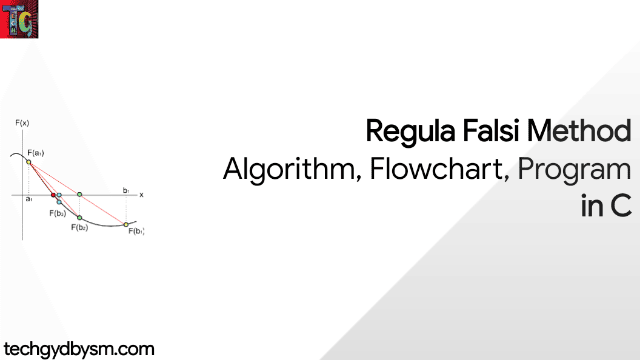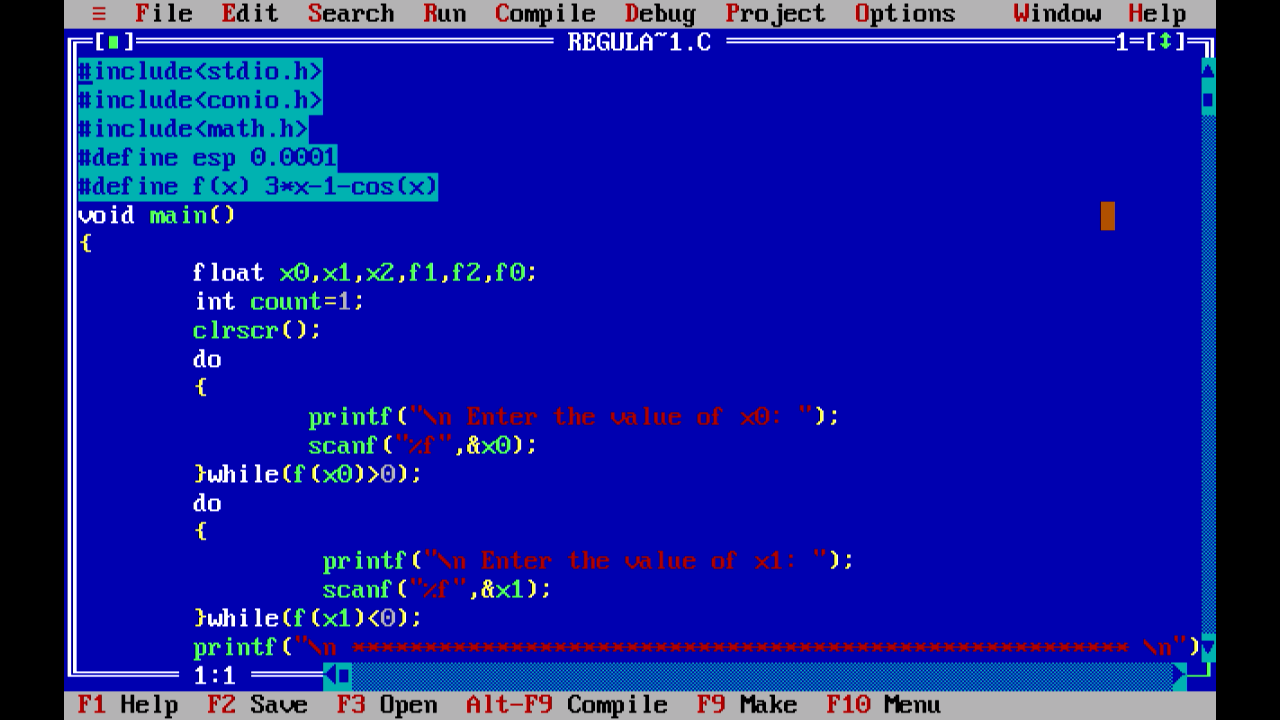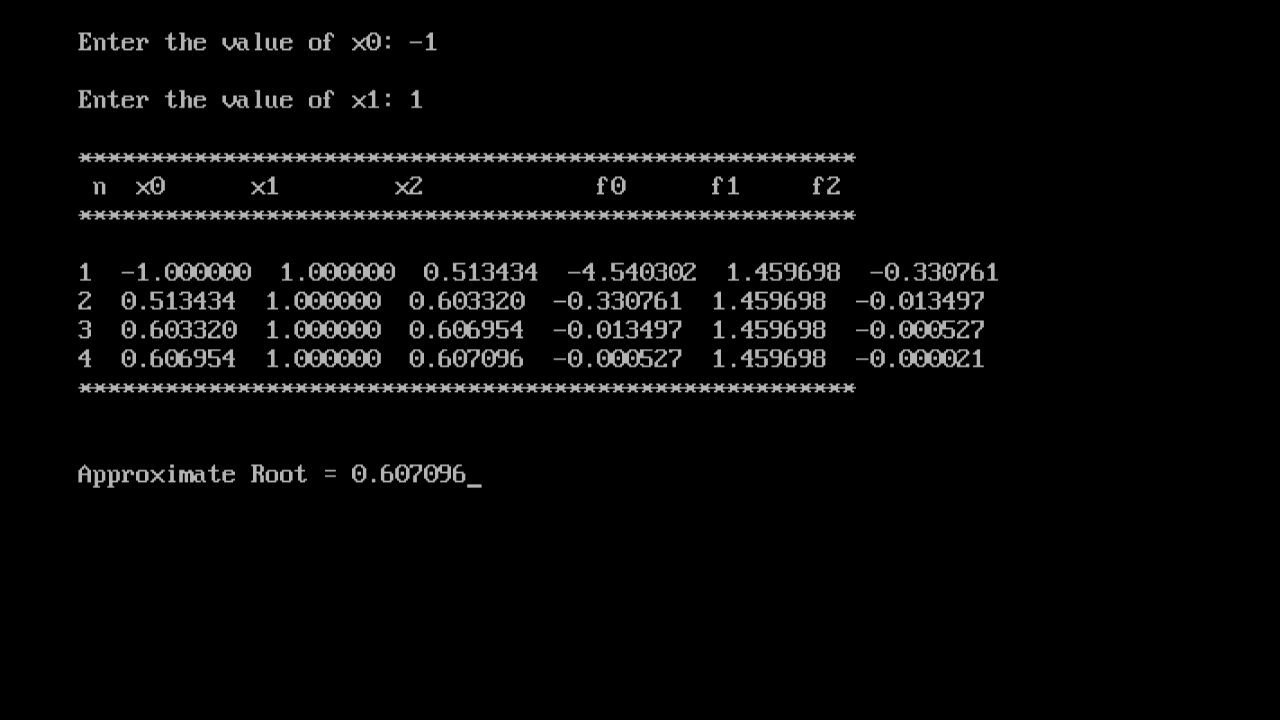# Regula Falsi Method: Algortihm, Flowchart, Program in C

C Programs has multiple application in several branches of science. One such application is in Numerical Analysis. The following article will guide you through the algorithm, flowchart and C Program to evaluate a Regula Falsi Method Numerical Problem using C Language.Regula Falsi Method in C

## What is Regula Falsi Method?

In this method, we first sufficiently choose a small interval [a0,b0], such that f(a0)f(b0)<0, by tabulation or graphical method, and which contains only one root a' (say) of f(x)=0, i.e., f'(x) maintains same sign in [a0,b0]. This method is based on the assumption that the graph of y=f(x) in small interval [a0,b0] can be represented by the chord joining (a0,f(a0)) and (b0,f(b0)). Therefore, the point x=a1=a0+h0 at which chord meets the x-axis gives us an approximate value of the root a' of the equation f(x)=0.

Thus, we obtain two intervals [a0,x1] and [x1,b0], one of which must contain the root a', depending upon the conditions f(a0)f(a')<0 or f(x1).f(b0)<0. If f(x1).f(b0)<0, then a' lies in the interval [x1,b0], which we rename as [a1,b1]. Again we consider that the graph of y=f(x) in [a1,b1] as the chord joining (a1,f(a1)) and (b1,f(b1)), thus, the point of intersection of the chord with the x-axis (say) x2=a1+h1 gives us an approximate value of the root a' and x2 is called second approximation of the root a'. Proceeding in this manner, we shall get a sequence {x1,x2,x3,...,xn}. Each member of which is the successive approximation of the exact root a' of the equation f(x)=0, geometrically.

## Computation Scheme

1. Find an interval [a0,b0], where f(a0).f(b0)<0 and f'(x) maintains the same sign.

2. Write n (number of iterations), an, bn, f(an), f(bn), hn={|f(an)|(bn-an)}/{|f(an)|+|f(bn)|} , xn+1=an+hn and f(xn+1) in a row.

3. Insert, +ve or -ve sign with an, as an(+) or an(-) according as f(a0)>0 or f(a0)<0 and -ve or +ve sign with bn as bn(-) or bn(+) according as f(b0)<0 or f(b0)>0.

4. In the (r+1)th iteration, write xr+1, in the column of an(+) if f(xr+1)>0 and keep br fixed in the column bn(-). Otherwise, write xr+1 in the column of bn(-) if f(xr+1)<0 and keep ar fixed in the column of an(+).

## Algorithm

STEP 1: Start
STEP 2: Read values of x0, x1 and e

Here x0 and x1 are the two initial guesses e is the degree of accuracy or the absolute error i.e. the stopping criteria

STEP 3: Computer function values f(x0) and f(x1)

STEP 4: Check whether the product of f(x0) and f(x1) is negative or not. If it is positive take another initial guesses. If it is negative then goto STEP 5.

STEP 5: Determine: x2 = [x0*f(x1) – x1*f(x0)] / (f(x1) – f(x0))

STEP 6: Check whether the product of f(x1) and f(x) is negative or not. If it is negative, then assign x0 = x2; If it is positive, assign x1 = x2;

STEP 7: Check whether the value of f(x) is greater than 0.00001 or not. If yes, goto STEP 5. If no, goto STEP 8.

Here the value 0.00001 is the desired degree of accuracy, and hence the stopping criteria.

STEP 8: Display the root as x.

STEP 9: Stop.

## FlowchartRegular Falsi Flowchart

## Sample Program

WAP in C to find the root of the equation 3x-cos(x)-1=0 using Method of Regular Falsi.

#### Code:

``````#include<stdio.h>
#include<conio.h>
#include<math.h>            /*included header files*/
#define esp 0.0001          /*defining error term*/
#define f(x) 3*x-1-cos(x)   /*defining the function*/
void main()
{
float x0,x1,x2,f1,f2,f0;
int count=1;
clrscr();
do
{
printf("\n Enter the value of x0: ");
scanf("%f",&x0);           /*loop to get x0*/
}while(f(x0)>0);
do
{
printf("\n Enter the value of x1: ");
scanf("%f",&x1);          /*loop to get x1*/
}while(f(x1)<0);
printf("\n ****************************************************** \n");
printf("  n  x0\t    x1\t       x2\t       f0\t       f1\t        f2");
printf("\n ****************************************************** \n");                 /*formatting the output*/
do
{
f0=f(x0);
f1=f(x1);
x2=x0-((f0*(x1-x0))/(f1-f0));         /*working formula loop*/
f2=f(x2);
printf("\n %d  %f  %f  %f  %f  %f  %f",count,x0,x1,x2,f0,f1,f2);
if(f0*f2<0)
x1=x2;
else
x0=x2;
count++;
}while(fabs(f2)>esp);
printf("\n ****************************************************** \n");
printf("\n\n Approximate Root = %f",x2);  /*final answer*/
getch();
}``````

#### Compiler Window:Compiler Window

#### Output Window:Output Screen

## Video Tutorial:

Feel free to comment below if you are facing problems while executing this program. Visit again soon.